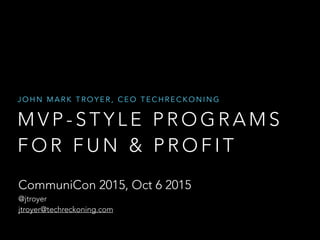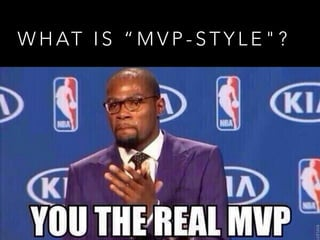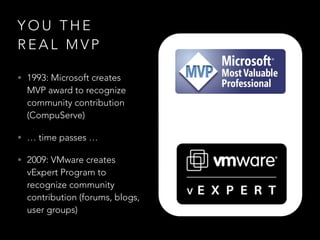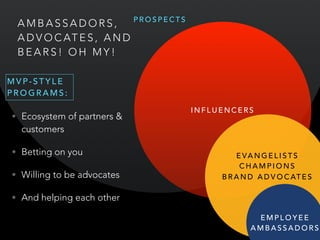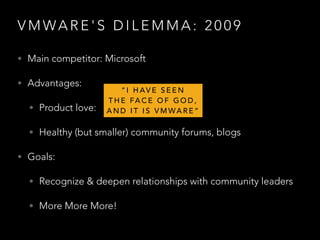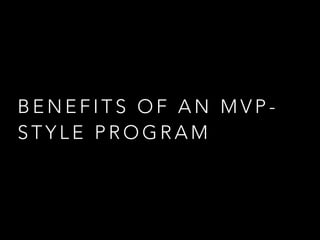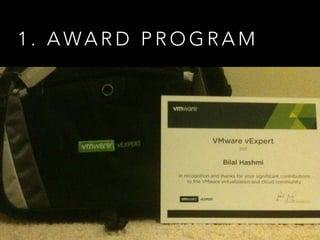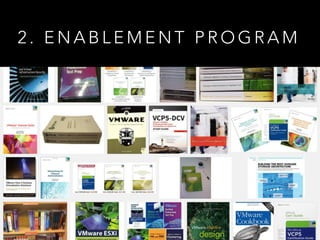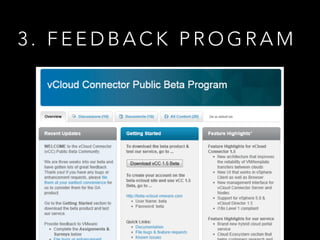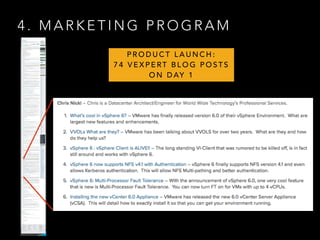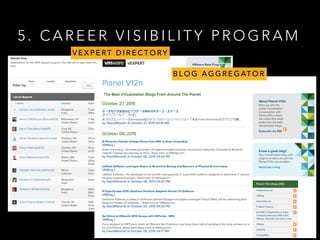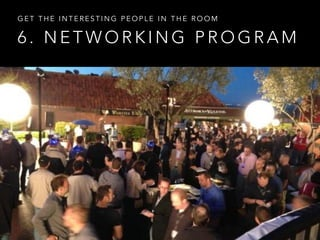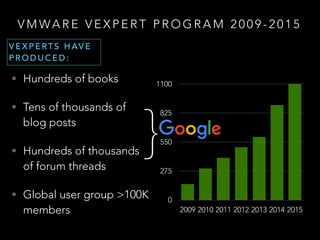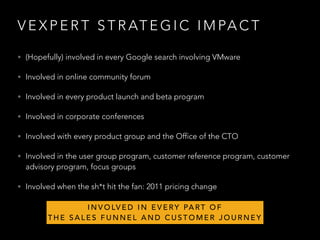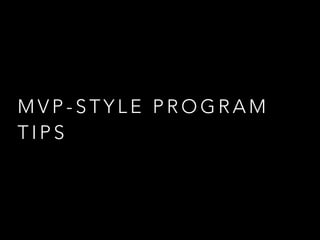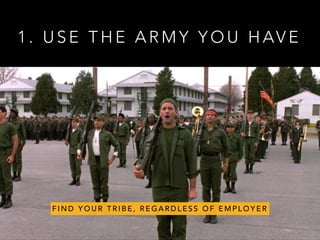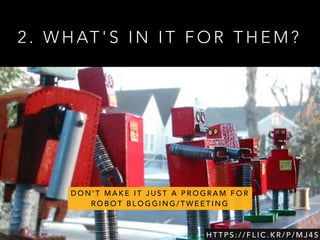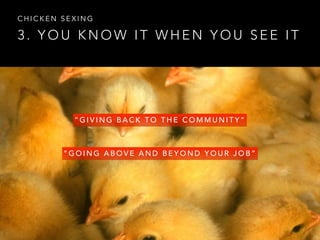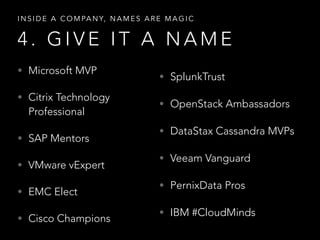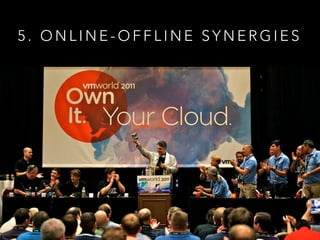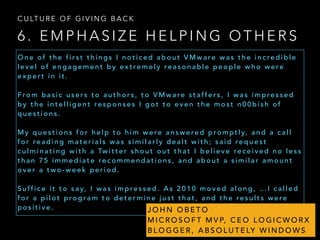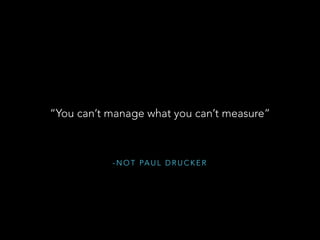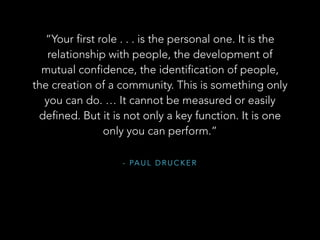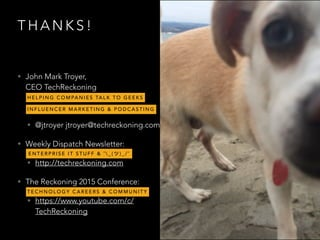1 de 24INCLUSIVE TRADE: THE RISE OF FAB LABSMartina F. FerracaneBecoming a Better Developer #WCA2Brian RichardsManufacturing the future: Industry 4.0Martina F. FerracaneSouth Africa & Data FlowsMartina F. FerracaneBuilding Multi-Site in Joomlabrian teemanSilicon Valley CultureTommaso Di Bartolo

### MVP-Style Influencer Programs for Fun & Profit

1. M V P - S T Y L E P R O G R A M S F O R F U N & P R O F I T J O H N M A R K T R O Y E R , C E O T E C H R E C K O N I N G CommuniCon 2015, Oct 6 2015 @jtroyer jtroyer@techreckoning.com
2. W H AT I S “ M V P - S T Y L E " ?
3. Y O U T H E R E A L M V P • 1993: Microsoft creates MVP award to recognize community contribution (CompuServe) • … time passes … • 2009: VMware creates vExpert Program to recognize community contribution (forums, blogs, user groups)
4. P R O S P E C T S I N F L U E N C E R S B R A N D A D V O C AT E S A M B A S S A D O R S , A D V O C AT E S , A N D B E A R S ! O H M Y ! • Ecosystem of partners & customers • Betting on you • Willing to be advocates • And helping each other E M P L O Y E E A M B A S S A D O R S M V P - S T Y L E P R O G R A M S : E VA N G E L I S T S C H A M P I O N S
5. V M WA R E ' S D I L E M M A : 2 0 0 9 • Main competitor: Microsoft • Advantages: • Product love: • Healthy (but smaller) community forums, blogs • Goals: • Recognize & deepen relationships with community leaders • More More More! “ I H AV E S E E N T H E FA C E O F G O D , A N D I T I S V M WA R E ”
6. B E N E F I T S O F A N M V P - S T Y L E P R O G R A M
7. 1 . A WA R D P R O G R A M
8. 2 . E N A B L E M E N T P R O G R A M
9. 3 . F E E D B A C K P R O G R A M
10. 4 . M A R K E T I N G P R O G R A M P R O D U C T L A U N C H : 7 4 V E X P E RT B L O G P O S T S O N D AY 1
11. 5 . C A R E E R V I S I B I L I T Y P R O G R A M V E X P E RT D I R E C T O RY B L O G A G G R E G AT O R
12. 6 . N E T W O R K I N G P R O G R A M G E T T H E I N T E R E S T I N G P E O P L E I N T H E R O O M
13. V M WA R E V E X P E R T P R O G R A M 2 0 0 9 - 2 0 1 5 • Hundreds of books • Tens of thousands of blog posts • Hundreds of thousands of forum threads • Global user group >100K members 0 275 550 825 1100 2009 2010 2011 2012 2013 2014 2015 V E X P E RT S H AV E P R O D U C E D : }
14. V E X P E R T S T R AT E G I C I M PA C T • (Hopefully) involved in every Google search involving VMware • Involved in online community forum • Involved in every product launch and beta program • Involved in corporate conferences • Involved with every product group and the Office of the CTO • Involved in the user group program, customer reference program, customer advisory program, focus groups • Involved when the sh*t hit the fan: 2011 pricing change I N V O LV E D I N E V E RY PA RT O F T H E S A L E S F U N N E L A N D C U S T O M E R J O U R N E Y
15. M V P - S T Y L E P R O G R A M T I P S
16. 1 . U S E T H E A R M Y Y O U H AV E F I N D Y O U R T R I B E , R E G A R D L E S S O F E M P L O Y E R
17. 2 . W H AT ' S I N I T F O R T H E M ? H T T P S : / / F L I C . K R / P / M J 4 S D O N ’ T M A K E I T J U S T A P R O G R A M F O R R O B O T B L O G G I N G / T W E E T I N G
18. 3 . Y O U K N O W I T W H E N Y O U S E E I T C H I C K E N S E X I N G “ G I V I N G B A C K T O T H E C O M M U N I T Y ” “ G O I N G A B O V E A N D B E Y O N D Y O U R J O B ”
19. 4 . G I V E I T A N A M E I N S I D E A C O M PA N Y, N A M E S A R E M A G I C • Microsoft MVP • Citrix Technology Professional • SAP Mentors • VMware vExpert • EMC Elect • Cisco Champions • SplunkTrust • OpenStack Ambassadors • DataStax Cassandra MVPs • Veeam Vanguard • PernixData Pros • IBM #CloudMinds
20. 5 . O N L I N E - O F F L I N E S Y N E R G I E S
21. 6 . E M P H A S I Z E H E L P I N G O T H E R S C U LT U R E O F G I V I N G B A C K O n e o f t h e f i r s t t h i n g s I n o t i c e d a b o u t V M w a re w a s t h e i n c re d i b l e l e v e l o f e n g a g e m e n t b y e x t re m e l y re a s o n a b l e p e o p l e w h o w e re e x p e r t i n i t . F ro m b a s i c u s e r s t o a u t h o r s , t o V M w a re s t a ff e r s , I w a s i m p re s s e d b y t h e i n t e l l i g e n t re s p o n s e s I g o t t o e v e n t h e m o s t n 0 0 b i s h o f q u e s t i o n s . M y q u e s t i o n s f o r h e l p t o h i m w e re a n s w e re d p ro m p t l y, a n d a c a l l f o r re a d i n g m a t e r i a l s w a s s i m i l a r l y d e a l t w i t h ; s a i d re q u e s t c u l m i n a t i n g w i t h a Tw i t t e r s h o u t o u t t h a t I b e l i e v e re c e i v e d n o l e s s t h a n 7 5 i m m e d i a t e re c o m m e n d a t i o n s , a n d a b o u t a s i m i l a r a m o u n t o v e r a t w o - w e e k p e r i o d . S u ff i c e i t t o s a y, I w a s i m p re s s e d . A s 2 0 1 0 m o v e d a l o n g , … I c a l l e d f o r a p i l o t p ro g r a m t o d e t e r m i n e j u s t t h a t , a n d t h e re s u l t s w e re p o s i t i v e . J O H N O B E T O M I C R O S O F T M V P, C E O L O G I C W O R X B L O G G E R , A B S O L U T E LY W I N D O W S
22. - N O T PA U L D R U C K E R “You can’t manage what you can’t measure”
23. - PA U L D R U C K E R “Your first role . . . is the personal one. It is the relationship with people, the development of mutual confidence, the identification of people, the creation of a community. This is something only you can do. … It cannot be measured or easily defined. But it is not only a key function. It is one only you can perform.”
24. T H A N K S ! • John Mark Troyer,   CEO TechReckoning • @jtroyer jtroyer@techreckoning.com • Weekly Dispatch Newsletter: • http://techreckoning.com • The Reckoning 2015 Conference: • https://www.youtube.com/c/ TechReckoning H E L P I N G C O M PA N I E S TA L K T O G E E K S E N T E R P R I S E I T S T U F F & ¯ _ ( ) _ / ¯ T E C H N O L O G Y C A R E E R S & C O M M U N I T Y I N F L U E N C E R M A R K E T I N G & P O D C A S T I N G